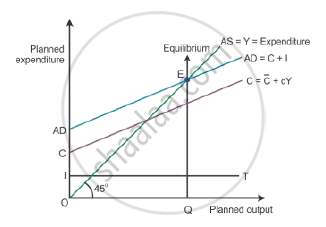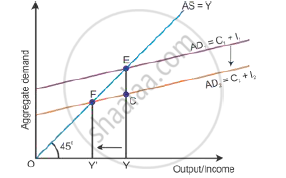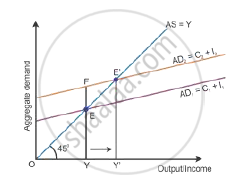# Explain National Income Equilibrium Through Aggregate Demand and Aggregate Supply. Use Diagram. Also Explain the Changes that Take Place in an Economy When the Economy is Not in Equilibrium - Economics

Explain national income equilibrium through aggregate demand and aggregate supply. Use diagram. Also explain the changes that take place in an economy when the economy is not in equilibrium

#### Solution

Aggregate demand and aggregate supply approach (AD and AS approach) Equilibrium level of income are attained only when aggregate demand is equal to aggregate supply. It is the level of output where producers plan to produce the amount of good is equal to consumers plan to purchase the amount of good. Thus, equilibrium is struck where planned output (AS) is equal to planned expenditure (AD) during a period of time.Deficient demand occurs in a situation when the aggregate demand is short of the aggregate supply corresponding to full employment in the economy. It leads to a fall in the general price level and results in deflation, i.e. AD < AS. Aggregate demand is shown by the AD curve and aggregate supply is shown by the AS curve (as shown in the diagram below). While the aggregate demand curve and the aggregate supply curve intersect each other, the full employment equilibrium is attained at Point E. OY is the full employment level of output, and EY is the aggregate demand at full employment level of output. If the aggregate demand decreases below the full employment level of output from EY to CY, then the economy will have deficient demand, (EY − CY = EC).Excess demand occurs in a situation when aggregate demand is more than aggregate supply corresponding to full employment. It leads to the reduction in inventories and inflation in the economy. This situation is considered an inflationary gap—the difference between aggregate demand beyond full employment and aggregate demand at full employment. Aggregate demand is the AD curve and aggregate supply is the AS curve (as shown in the diagram below). While the aggregate demand curve and the aggregate supply curve intersect each other, the full employment equilibrium is attained at Point E. OY is the full employment level of output, and EY is the aggregate demand at full employment level of output. If the aggregate demand increases beyond the full employment level of output from EY to FY, then the economy will have excess demand (FY − EY = FE).Concept: Concept of Aggregate Demand and Aggregate Supply
Is there an error in this question or solution?